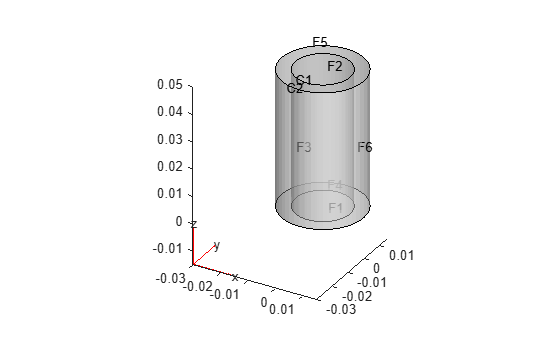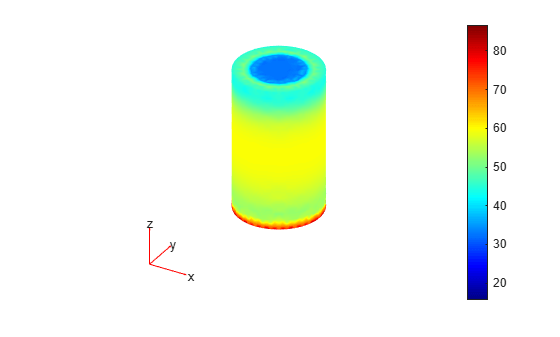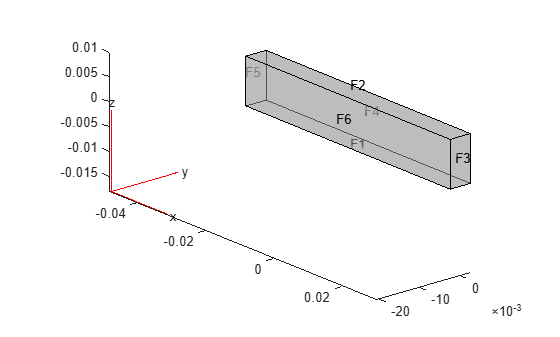# evaluatePrincipalStress

Evaluate principal stress at nodal locations

## Syntax

``pStress = evaluatePrincipalStress(structuralresults)``

## Description

example

````pStress = evaluatePrincipalStress(structuralresults)` evaluates principal stress at nodal locations using stress values from `structuralresults`. For transient and frequency response structural models, `evaluatePrincipalStress` evaluates principal stress for all time- and frequency-steps, respectively.```

## Examples

collapse all

Solve a static structural model representing a bimetallic cable under tension, and compute octahedral shear stress.

Create a structural model.

`structuralmodel = createpde('structural','static-solid');`

Create a geometry and include it in the model. Plot the geometry.

```gm = multicylinder([0.01,0.015],0.05); structuralmodel.Geometry = gm; pdegplot(structuralmodel,'FaceLabels','on', ... 'CellLabels','on', ... 'FaceAlpha',0.5)```Specify Young's modulus and Poisson's ratio for each metal.

```structuralProperties(structuralmodel,'Cell',1,'YoungsModulus',110E9, ... 'PoissonsRatio',0.28); structuralProperties(structuralmodel,'Cell',2,'YoungsModulus',210E9, ... 'PoissonsRatio',0.3);```

Specify that faces 1 and 4 are fixed boundaries.

`structuralBC(structuralmodel,'Face',[1,4],'Constraint','fixed');`

Specify the surface traction for faces 2 and 5.

```structuralBoundaryLoad(structuralmodel,'Face',[2,5], ... 'SurfaceTraction',[0;0;100]);```

Generate a mesh and solve the problem.

```generateMesh(structuralmodel); structuralresults = solve(structuralmodel)```
```structuralresults = StaticStructuralResults with properties: Displacement: [1x1 FEStruct] Strain: [1x1 FEStruct] Stress: [1x1 FEStruct] VonMisesStress: [22281x1 double] Mesh: [1x1 FEMesh] ```

Evaluate the principal stress at nodal locations.

`pStress = evaluatePrincipalStress(structuralresults);`

Use the principal stress to evaluate the first and second invariant of stress.

```I1 = pStress.s1 + pStress.s2 + pStress.s3; I2 = pStress.s1.*pStress.s2 + ... pStress.s2.*pStress.s3 + ... pStress.s3.*pStress.s1; tauOct = sqrt(2*(I1.^2 -3*I2))/3; pdeplot3D(structuralmodel,'ColorMapData',tauOct)```Evaluate the principal stress and octahedral shear stress in a beam under a harmonic excitation.

Create a transient dynamic model for a 3-D problem.

`structuralmodel = createpde('structural','transient-solid');`

Create the geometry and include it in the model. Plot the geometry.

```gm = multicuboid(0.06,0.005,0.01); structuralmodel.Geometry = gm; pdegplot(structuralmodel,'FaceLabels','on','FaceAlpha',0.5) view(50,20)```Specify Young's modulus, Poisson's ratio, and the mass density of the material.

```structuralProperties(structuralmodel,'YoungsModulus',210E9, ... 'PoissonsRatio',0.3, ... 'MassDensity',7800);```

Fix one end of the beam.

`structuralBC(structuralmodel,'Face',5,'Constraint','fixed');`

Apply a sinusoidal displacement along the y-direction on the end opposite the fixed end of the beam.

```structuralBC(structuralmodel,'Face',3,... 'YDisplacement',1E-4,... 'Frequency',50);```

Generate a mesh.

`generateMesh(structuralmodel,'Hmax',0.01);`

Specify the zero initial displacement and velocity.

`structuralIC(structuralmodel,'Displacement',[0;0;0],'Velocity',[0;0;0]);`

Solve the model.

```tlist = 0:0.002:0.2; structuralresults = solve(structuralmodel,tlist);```

Evaluate the principal stress in the beam.

`pStress = evaluatePrincipalStress(structuralresults);`

Use the principal stress to evaluate the first and second invariants.

```I1 = pStress.s1 + pStress.s2 + pStress.s3; I2 = pStress.s1.*pStress.s2 + ... pStress.s2.*pStress.s3 + ... pStress.s3.*pStress.s1;```

Use the stress invariants to compute the octahedral shear stress.

`tauOct = sqrt(2*(I1.^2 -3*I2))/3;`

Plot the results.

```figure pdeplot3D(structuralmodel,'ColorMapData',tauOct(:,end))```## Input Arguments

collapse all

Solution of the structural analysis problem, specified as a `StaticStructuralResults`, `TransientStructuralResults`, or `FrequencyStructuralResults` object. Create `structuralresults` by using the `solve` function.

Example: ```structuralresults = solve(structuralmodel)```

## Output Arguments

collapse all

Principal stress at the nodal locations, returned as a structure array.

## Version History

Introduced in R2017b